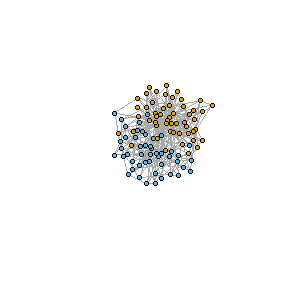# Other Functions

library(clustAnalytics)

### reduced_mutual_information

The package includes an implementation of Newman’s Reduced Mutual Information (RMI) , a version of the mutual information that is corrected for chance.

data(karate, package="igraphdata")
c1 <- membership(cluster_louvain(karate))
c2 <- V(karate)$Faction reduced_mutual_information(c1, c2, method="approximation2") #>  0.5135699 Just as with the standard mutual information, the RMI can be normalized as well: reduced_mutual_information(c1, c2, method="approximation2", normalized=TRUE) #>  0.6621045 ### barabasi_albert_blocks The barabasi_albert_blocks function produces scale-free graphs using extended versions of the Barabási-Albert model that include a community structure. It generates the graph by iteratively adding vertices to an initial graph and joining them to the existing vertices using preferential attachment (existing higher degree vertices are more likely to receive new edges). Additionally, vertices are assigned labels indicating community membership, and the probability of one vertex connecting to another is affected by their community memberships according to a fitness matrix B (if a new vertex belongs to community i, the probability of connecting to a vertex of community j is proportional to B_ij). The parameters that need to be set are m the number of new edges per step, the vector p of label probabilities, the fitness matrix B (with the same dimensions as the length of p), and t_max the final graph order. The initial graph G0 can be set manually, but if not, an appropriate graph will be generated with m edges per vertex, labels sampled from p, and edge probabilities proportional B. There are two variants of the model. If type="Hajek", new edges are connected with preferential attachment to any existing vertex but using the appropriate values of B as weights (see ). If type="block_first", new edges are connected first to a community with probability proportional to the values of B, and then a vertex is chosen within that community with regular preferential attachment. In this case, the resulting degree distribution is scale-free (see ). This is a simple example with just two communities and a graph of order 100 and size 400: B <- matrix(c(1, 0.2, 0.2, 1), ncol=2) G <- barabasi_albert_blocks(m=4, p=c(0.5, 0.5), B=B, t_max=100, type="Hajek", sample_with_replacement = FALSE) plot(G, vertex.color=(V(G)$label), vertex.label=NA, vertex.size=10)### apply_subgraphs

The apply_subgraphs function is used internally in the package, but has also been made available to the user because it can be very convenient when working with clusters. It simply calls a function f on each of the communities of a graph (treated as it’s own igraph object), acting as a wrapper for the vapply function. The communities are given as a membership vector com.

For a very simple example, we call it to obtain the order of each of the factions of the karate club graph:

data(karate, package="igraphdata")
apply_subgraphs(g=karate, com=V(karate)\$Faction, f=gorder)
#>  16 18